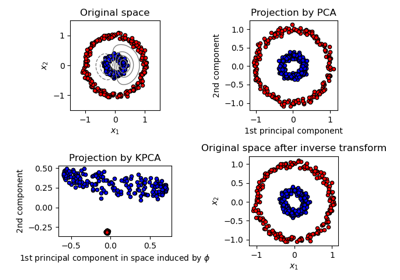# sklearn.decomposition.KernelPCA¶

class sklearn.decomposition.KernelPCA(n_components=None, *, kernel='linear', gamma=None, degree=3, coef0=1, kernel_params=None, alpha=1.0, fit_inverse_transform=False, eigen_solver='auto', tol=0, max_iter=None, remove_zero_eig=False, random_state=None, copy_X=True, n_jobs=None)[source]

Kernel Principal component analysis (KPCA)

Non-linear dimensionality reduction through the use of kernels (see Pairwise metrics, Affinities and Kernels).

Read more in the User Guide.

Parameters
n_componentsint, default=None

Number of components. If None, all non-zero components are kept.

kernel“linear” | “poly” | “rbf” | “sigmoid” | “cosine” | “precomputed”

Kernel. Default=”linear”.

gammafloat, default=1/n_features

Kernel coefficient for rbf, poly and sigmoid kernels. Ignored by other kernels.

degreeint, default=3

Degree for poly kernels. Ignored by other kernels.

coef0float, default=1

Independent term in poly and sigmoid kernels. Ignored by other kernels.

kernel_paramsmapping of string to any, default=None

Parameters (keyword arguments) and values for kernel passed as callable object. Ignored by other kernels.

alphaint, default=1.0

Hyperparameter of the ridge regression that learns the inverse transform (when fit_inverse_transform=True).

fit_inverse_transformbool, default=False

Learn the inverse transform for non-precomputed kernels. (i.e. learn to find the pre-image of a point)

eigen_solverstring [‘auto’|’dense’|’arpack’], default=’auto’

Select eigensolver to use. If n_components is much less than the number of training samples, arpack may be more efficient than the dense eigensolver.

tolfloat, default=0

Convergence tolerance for arpack. If 0, optimal value will be chosen by arpack.

max_iterint, default=None

Maximum number of iterations for arpack. If None, optimal value will be chosen by arpack.

remove_zero_eigboolean, default=False

If True, then all components with zero eigenvalues are removed, so that the number of components in the output may be < n_components (and sometimes even zero due to numerical instability). When n_components is None, this parameter is ignored and components with zero eigenvalues are removed regardless.

random_stateint, RandomState instance, default=None

Used when eigen_solver == ‘arpack’. Pass an int for reproducible results across multiple function calls. See Glossary.

New in version 0.18.

copy_Xboolean, default=True

If True, input X is copied and stored by the model in the X_fit_ attribute. If no further changes will be done to X, setting copy_X=False saves memory by storing a reference.

New in version 0.18.

n_jobsint or None, optional (default=None)

The number of parallel jobs to run. None means 1 unless in a joblib.parallel_backend context. -1 means using all processors. See Glossary for more details.

New in version 0.18.

Attributes
lambdas_array, (n_components,)

Eigenvalues of the centered kernel matrix in decreasing order. If n_components and remove_zero_eig are not set, then all values are stored.

alphas_array, (n_samples, n_components)

Eigenvectors of the centered kernel matrix. If n_components and remove_zero_eig are not set, then all components are stored.

dual_coef_array, (n_samples, n_features)

Inverse transform matrix. Only available when fit_inverse_transform is True.

X_transformed_fit_array, (n_samples, n_components)

Projection of the fitted data on the kernel principal components. Only available when fit_inverse_transform is True.

X_fit_(n_samples, n_features)

The data used to fit the model. If copy_X=False, then X_fit_ is a reference. This attribute is used for the calls to transform.

References

Kernel PCA was introduced in:

Bernhard Schoelkopf, Alexander J. Smola, and Klaus-Robert Mueller. 1999. Kernel principal component analysis. In Advances in kernel methods, MIT Press, Cambridge, MA, USA 327-352.

Examples

>>> from sklearn.datasets import load_digits
>>> from sklearn.decomposition import KernelPCA
>>> transformer = KernelPCA(n_components=7, kernel='linear')
>>> X_transformed = transformer.fit_transform(X)
>>> X_transformed.shape
(1797, 7)


Methods

 fit(X[, y]) Fit the model from data in X. fit_transform(X[, y]) Fit the model from data in X and transform X. get_params([deep]) Get parameters for this estimator. Transform X back to original space. set_params(**params) Set the parameters of this estimator. Transform X.
__init__(n_components=None, *, kernel='linear', gamma=None, degree=3, coef0=1, kernel_params=None, alpha=1.0, fit_inverse_transform=False, eigen_solver='auto', tol=0, max_iter=None, remove_zero_eig=False, random_state=None, copy_X=True, n_jobs=None)[source]

Initialize self. See help(type(self)) for accurate signature.

fit(X, y=None)[source]

Fit the model from data in X.

Parameters
Xarray-like, shape (n_samples, n_features)

Training vector, where n_samples in the number of samples and n_features is the number of features.

Returns
selfobject

Returns the instance itself.

fit_transform(X, y=None, **params)[source]

Fit the model from data in X and transform X.

Parameters
Xarray-like, shape (n_samples, n_features)

Training vector, where n_samples in the number of samples and n_features is the number of features.

Returns
X_newarray-like, shape (n_samples, n_components)
get_params(deep=True)[source]

Get parameters for this estimator.

Parameters
deepbool, default=True

If True, will return the parameters for this estimator and contained subobjects that are estimators.

Returns
paramsmapping of string to any

Parameter names mapped to their values.

inverse_transform(X)[source]

Transform X back to original space.

Parameters
Xarray-like, shape (n_samples, n_components)
Returns
X_newarray-like, shape (n_samples, n_features)

References

“Learning to Find Pre-Images”, G BakIr et al, 2004.

set_params(**params)[source]

Set the parameters of this estimator.

The method works on simple estimators as well as on nested objects (such as pipelines). The latter have parameters of the form <component>__<parameter> so that it’s possible to update each component of a nested object.

Parameters
**paramsdict

Estimator parameters.

Returns
selfobject

Estimator instance.

transform(X)[source]

Transform X.

Parameters
Xarray-like, shape (n_samples, n_features)
Returns
X_newarray-like, shape (n_samples, n_components)

## Examples using sklearn.decomposition.KernelPCA¶